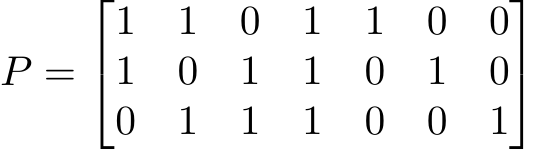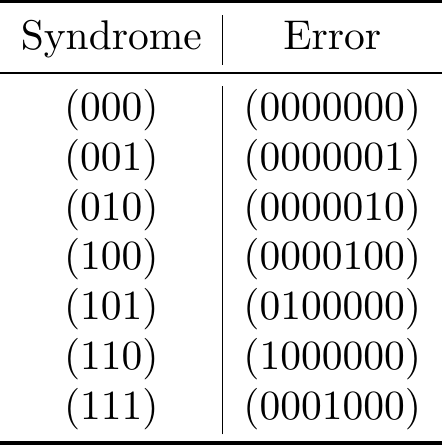# Coding Theory: Hamming and Golay codes with Huffman Optimisation

### Overview

Error correcting codes (ECC) are used for controlling errors over noisy channels. They work by adding extra data to the message as redundant binary. This redundant binary allows the receiver to detect and correct a finite amount of errors. ECC’s are very important as they provide an alternate option of error correcting than the retransmission of the message. In some cases, sending a message may take hours and cost a significant amount of money so the retransmission of the message isn’t viable. ECC is also applied when transmitting to multiple receivers in multicast and also for one way communication links.

ECC’s were first introduced by Richard Hamming during the 1940’s who invented the first error correction code, the Hamming code.

### Theory

All error correcting codes are based off a unique [n, k, d] structure, where n, k d ϵ N. ‘n’ represents the dimension of the encoded message with added redundant information – the code word. ‘k’ represents the dimension of the original message. ‘d’ represents the minimum distance between any two code words. Distance is defined as the number of bits that differ between codewords. For instance, the distance between ‘000’ and ‘111’ is 3.

An example of an error correcting code is the [3, 1, 3] repetition code, where ‘0’ -> ‘000’ and ‘1’ -> ‘111’. These are the only two code words.

Naturally, there are some obvious constraints for any code following the [n, k, d] structure. For example, n > k. If this were not true, it would be impossible to correct errors. Also, d ≤ n since n is the maximum number of possible bits.

If d is expressed as d = 2e + 1 rounding down, the number of errors that can be corrected is ‘e’. For instance, if d = 3, then e = 1. This is the basis off which error-correcting codes function. (diagram)

Linear codes can be represented using a generating matrix. A generating matrix takes a vector of length k and transforms it to a vector of length n. Further, each generating matrix has its own parity check matrix. This matrix takes any vector V of length n and outputs the zero vector if V is a code word. Otherwise, the matrix will output a vector of length n – k, which is call the syndrome.

For a linear code that can correct up to one error, the column of the parity check matrix that is equal to the syndrome means that the corresponding column of the vector V contains an error.

### Hamming Code

The Hamming code (7,4,3) is a linear block code that acts on a block of 4 bits and produces 7 bits of output. This code can detect and correct only single bit errors. The hamming code (7,4,3) adds 3 redundant bits.

Hamming error correcting uses a generator matrix, parity check matrix and syndromes to detect and correct errors.

The generator matrix seen below has rows which form the basis for the linear hamming code (7,4,3). The code words are linear combinations of this matrix’s rows. This generator matrix gives you a code word whenever it is multiplied by a word.Say that you have a linear code with parity matrix P and you were trying to send the code word x but received z = x + e, where e is some error. Performing the matrix multiplication gives you PzT = P(x + e)T = PxT + PeT. However, as x is in the kernel of P we have that PxT = 0 and thus that PzT = PeT = s. Using a pre-computed lookup table, we are able to find that s corresponds to the error e and thus we are able to find x as x = z − e. This method is known as syndrome decoding. The parity matrix and syndrome list for the hamming (7,4,3) code is below:An example of using the Hamming code is below:

Let’s say you wanted to encode m = (0110) with the [7, 4, 3] code. You multiply it by G to get the code word. mG = (0110110) = c Say that when this is transmitted, you receive z = (1110110). Multiplying this by the parity matrix gives you: P zT = (110) Using the syndrome lookup table, we can see that this means an error occurred in the first bit. Thus, when we correct the error we get z − (1000000) = (0110110) = c. As the generator matrix is in the form [I4|P], the first 4 components of the vector correspond to the original message. Thus, we can just take those first four components in order to decode the message.

Hamming code is being used in memory chips and satellite communication today. Initially, Richard Hamming during the 1950s to automatically correct errors caused by punch card readers.

### Golay

The Extended Golay Code (24, 12, 8) is a linear block code that acts on blocks of 12 bits and produces 24 bit blocks as output. It can detect and correct up to three errors.

The Perfect Golay Code (23, 12, 7) is a linear block code that can also detect and correct up to three errors. This means that the balls of radius 3 around code words form a partition of the vector space. In other words, no matter what error occurs, you will always be able to decode the received message. Note that it will not necessarily be a correct decoding.

The generator matrix for the Extended Golay Code is shown below. The Extended Goaly Code is a self-dual code, meaning that its parity check matrix is the same as its generator matrix. There are 2325 possible syndromes for the Extended Golay Code.The Golay codes were developed by Marcel J. E. Golay and were named in honour of him. A notable use of them was when NASA used the Extended Golay Code in the Voyage 1 and 2 spacecraft.

### Huffman

Huffman code is not an error correcting code. Rather, it is a code commonly used for lossless data compression to improve efficiency. Huffman code was created by David A. Huffman in 1951 to find the most efficient binary code. He could not prove that any code was the most efficient, so instead he created this code and quickly proved it as the most efficient.

Huffman code is based upon the idea of a frequency-sorted binary tree. It takes the most common character in a message and assigns it the least number of bits. Going on like this, the least common character is assigned the greatest number of bits. This allows a message to contain fewer bits when compared to say using ASCII characters which are each 8 bits long.

While the Hamming and Golay codes are block codes, the Huffman code is not. To ensure that messages are decoded correctly, each code word is not a prefix of any other code word. This allows a receiver to recognise the beginning and end of code words without them being the same length.

Although Huffman code increases the efficiency of a message, it can be problematic when used in conjunction with an error correcting code and a noisy channel.

For instance, say a message that has been encoded using both Huffman and Hamming code has been partially corrupted. When decoding the message, if the Hamming code does not correct all errors, a part of the message may be decoded earlier or later than it should be. If this happens, the next letter won't be decoded correctly, and the error can propagate through the message. In block code each code word length is known, so they can be skipped if there is an error.

So, while using a Huffman code reduces the message size and thus potential for it to be corrupted, it can make some messages unable to decode if corrupted.

Your message when encoded with the Hamming Code is:

Your message when encoded with the Golay Code is:

The Hamming Code when affected by noise is:

The Golay Code when affected by noise is:

Your message when decoded with the Hamming Code is:

Your message when decoded with the Golay Code is:

The number of errors with the Hamming Code is:

The number of errors with the Golay Code is: